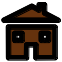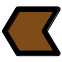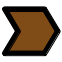# Friction noise of rough surface

Experiment on friction noise Dependance of friction noise with roughness and sliding speed Dependance of friction noise with contact area An incredible experiment with sugar lumps Direct numerical simulation

### Experiment on friction noise.

When rubbing two rough surfaces against each other, a particular friction noise is generated. The fact that surfaces are rough induces many shocks between antagonist asperitites. These shocks are responsible of the vibration of solids which, in turn radiates the sound. This is an example of phenomenon which involve the dynamics of multicontact interfaces.The following experiment is designed to measure the empirical laws of roughness noise versus, roughness of surfaces, material of solids, sliding speed and contact area.Experiment to measure empirical laws of friction noise.

### Dependance of friction noise with roughness and sliding speed.

The friction sound is found to have an increasing level with both, roughness of surfaces and sliding speed. The following figures show an example of empirical laws obtained with our experiment. It can be shown that the evolution follows a log-log law.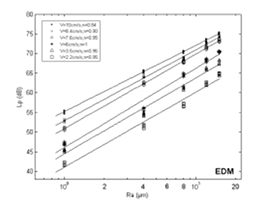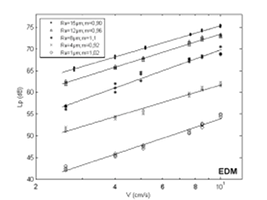H. Ben Abdelounis, A. Le Bot, J. Perret-Liaudet, H. Zahouani. "An experimental study on roughness noise of dry rough flat surfaces," Wear, vol. 268, pages 335-345, 2010.

B. Stoimenov, S. Maruyama, K. Adachi, K. Kato, The roughness effect on the frequency of frictional sound, Tribology International, vol. 40, p. 659-664, 2007.

### Dependance of friction noise with contact area.

Curiously, the dependance of friction sound with the contact area (in fact the number of sliders with same nominal area) is not proportional. Two regimes are found. For small contact area, the proportionality applies (slope ~10 dB/decade). In this regime, the sound produced by sliders is added explaning the linear law. But for large area, the sound is constant and does not depend on the number of sliders (slope ~0 dB/decade). This is a strange result since there is apparently no reason for which the addiditivity of sound would no longer apply.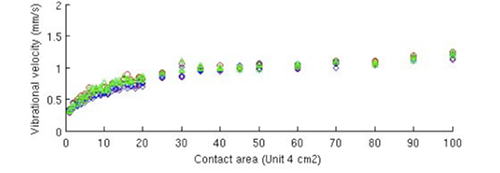Evolution of friction sound with contact area.

A. Le Bot and E. Bou Chakra. "Measurement of friction noise versus contact area of rough surfaces weakly loaded," Tribology Letters, vol. 37, pages 273-281, 2010.

### An incredible experiment with sugar lumps.

Take some sugar lumps and rubb them on a large surface like a wood table for instance. Do you think that the friction sound will be stronger with a larger number of sugar lumps? Definitely yes (try it!). If the number of lumps is multiplied by ten, then the sound is ten times much strong (10 dB/decade). But if you do again the experiment on a drum, you will observe that the sound is almost constant and do not depend on the number of lumps (do it!). This is an illustration of the fact that the sound power is not proportional to contact area.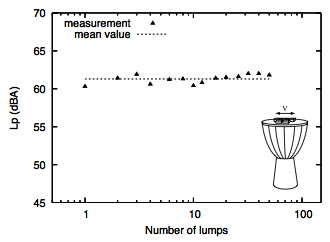Friction sound do not depend on the number of sugar lumps on a drum membrane.

A. Le Bot, E. Bou Chakra and G. Michon. "Dissipation of vibration in rough contact," Tribology Letters, vol. 41, pages 47-53, 2011.

### Direct numerical simulation.

The direct numerical simulation of the sliding of deformable rough surfaces is possible in principle but very difficult in practice. The size of surface asperities being of order of micrometer, the number of contacts per seconds is very large and a very large computer is required to perform these computations. We did it and we observed that micro-impacts occuring in the interface generate vibration of surfaces which, in turn, is responsible of the sound.

Friction sound is produced by impacts between antagonist asperities.

V.H. Dang, J. Perret-Liaudet, J. Scheibert, A. Le Bot. "Direct numerical simulation of the dynamics of sliding rough surfaces," Computational mechanics, vol. 52, pages 1169-1183, 2013.